# Work and Wages - Quantitative Aptitude (MCQ) questions

Dear Readers, Welcome to Quantitative Aptitude Work and Wages questions and answers with explanation. These Work and Wages solved examples with shortcuts and tricks will help you learn and practice for your Placement Test and competitive exams like Bank PO, IBPS PO, SBI PO, RRB PO, RBI Assistant, LIC,SSC, MBA - MAT, XAT, CAT, NMAT, UPSC, NET etc.

After practicing these tricky Work and Wages multiple choice questions, you will be exam ready to deal with any objective type questions.

1)   Ram and Shyam have been given a task of painting a house for Rs. 800. With the help of Rita, they complete the job in just 3 days. Had Ram alone be doing the task, he would need 6 days. If Shyam alone would be doing the task, he would need 8 days. How much money will Rita get?
- Published on 08 May 17

a. Rs. 80
b. Rs. 150
c. Rs. 100
d. Rs. 125
 Answer  Explanation ANSWER: Rs. 100 Explanation: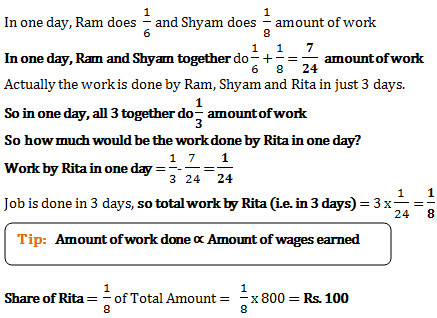2)   P and Q together earn Rs. 188 per day. Q and R together earn Rs. 152 per day. P, Q and R when working together earn Rs. 300 per day. How much does Q earn daily?
- Published on 08 May 17

a. Rs. 43
b. Rs. 56
c. Rs. 45
d. Rs. 40
 Answer  Explanation ANSWER: Rs. 40 Explanation: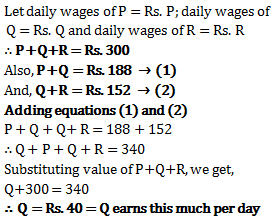3)   What will be share of Rajesh, if together Ramesh and Suresh complete only 7/11 of the task, and all three had been given the contract to finish the task for Rs. 1100?
- Published on 08 May 17

a. Rs. 350(4/11)
b. Rs. 200
c. Rs. 400
d. Rs. 650
 Answer  Explanation ANSWER: Rs. 400 Explanation: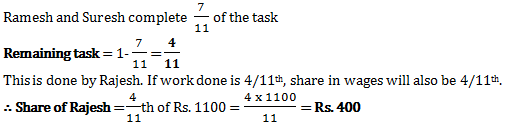4)   P and Q work together on a task for 5 days. They then leave and rest of the task is done by R in just 2 days. All the three together are paid Rs. 450. What will be R’s share out of the payment if P alone could do the task in 10 days and Q alone can do the task alone in 15 days?
- Published on 08 May 17

a. Rs. 100
b. Rs. 75
c. Rs. 225
d. Rs. 50
 Answer  Explanation ANSWER: Rs. 75 Explanation: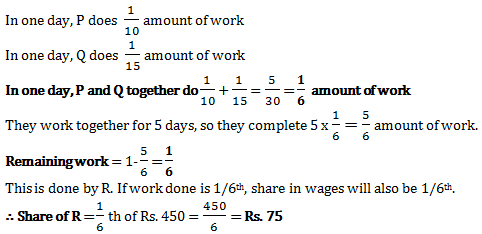5)   P and Q work together and get a payment of Rs. 1400. P can complete the work alone in just 30 days. But Q takes 40 days to complete the work alone. What is P’s share out of the wages they get?
- Published on 08 May 17

a. Rs. 800
b. Rs. 600
c. Rs. 1000
d. Rs. 700
 Answer  Explanation ANSWER: Rs. 800 Explanation: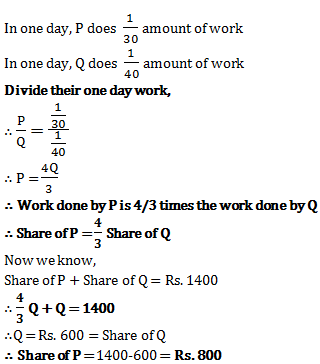6)   Johnny employs 8 workers to work for 6 hours per day. In total he pays them Rs. 630 for a week. How much should Johnny pay 18 workers working 4 hours per day for a week?
- Published on 03 May 17

a. Rs. 945
b. Rs. 645
c. Rs. 630
d. Rs. 1050
 Answer  Explanation ANSWER: Rs. 945 Explanation: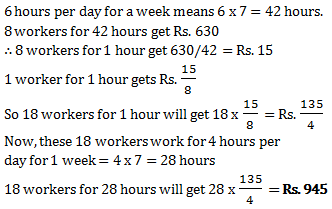7)   The amount of money with which A’s wage can be paid for 18 days, when A is working alone, is enough for paying B’s wage for 12 days, when B is working alone. If A and B start working together, then the same amount would be enough for wages of both of them for how many days?
- Published on 13 Apr 17

a. 36/5 days
b. 5/36 days
c. 15 days
d. 6 days
 Answer  Explanation ANSWER: 36/5 days Explanation: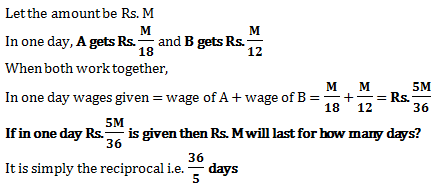8)   P, Q and R get Rs. 10800 for doing a work in 18 days. P and R get Rs. 3760 for doing the same work in 10 days, while Q and R get Rs. 6080 for doing the same work in 20 days. Find the amount received by R per day?
- Published on 17 Mar 17

a. Rs. 95
b. Rs. 126
c. Rs. 80
d. Rs. 200
 Answer  Explanation ANSWER: Rs. 80 Explanation: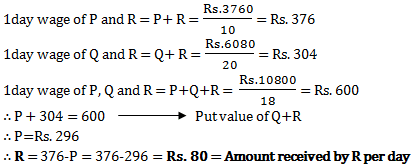9)   Wages of 44 women for 56 days comes to Rs.29568. How many men are needed for 47days to receive Rs. 16920, if the daily wages of a man being 5 times those of a woman?
- Published on 17 Mar 17

a. 9 men
b. 8 men
c. 6 men
d. 5 men
 Answer  Explanation ANSWER: 6 men Explanation: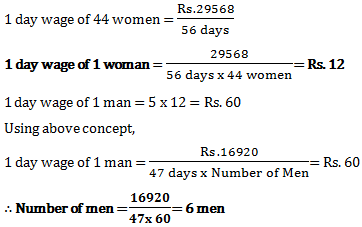10)   Total wages of 6 men, 4 women and 8 boys is Rs. 26. If the wages of 6 men is equal to that of 8 women and the wages of 4 women is equal to that of 6 boys, then find out the total wages of 8 men, 6 women and 4 boys?
- Published on 15 Mar 17

a. Rs. 32
b. Rs. 24
c. Rs. 25
d. Rs. 29
 Answer  Explanation ANSWER: Rs. 29 Explanation: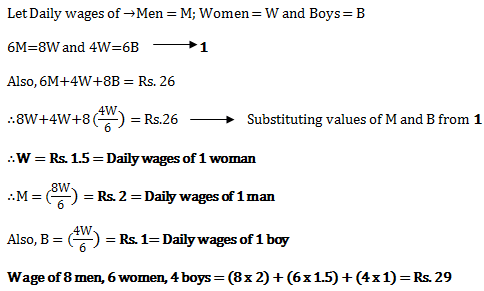1 2